# Difference between revisions of "Examples in mathematics"

Examples in mathematics have different flavor than examples in other disciplines. This is probably because definitions in mathematics are different from definitions in other disciplines (mathematical definitions are exact). Some common problems of deciding whether something is or is not an example do not appear in mathematics. Instead, there are other problems.

## Unit testing and examples

A common problem in math is that one comes in with some preconceived idea of what an object should "look like" which is different from what the definition says. In other words, there is a mismatch between one's intuitive notion and the definition.

Take the example of a definition of function. A function is some object that takes each object in some set to a unique object in another set. Someone who was not familiar with the formal definition might mistakenly think of a function as "something that is defined by a formula".

Is an example according to definition Is not an example according to definition
Is an example according to intuition An "obvious" example, or central example.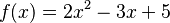$f(x) = 2x^2 - 3x + 5$ A surprising non-example.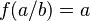$f(a/b) = a$ (i.e. a function that outputs the numerator of a fraction); a function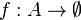$f : A \to \emptyset$ where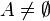$A \ne \emptyset$.
Is not an example according to intuition A surprising example.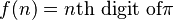$f(n) = n\text{th digit of}\pi$; the empty function;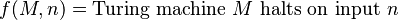$f(M,n) = \text{Turing machine }M\text{ halts on input }n$ An obvious non-example.

## Hierarchical nature of examples

Something can be considered "concrete" or "abstract" depending on the context. Consider a term like "metric space". One can give examples of metric spaces. On the other hand, a metric space is itself an example (of a structured space, of a topological space).